## 1 Introduction

The understanding of strongly correlated many-body systems like the two-dimensional Hubbard model remains an important challenge of contemporary condensed-matter physics . For this, it is desirable to gain profound understanding of two-body interactions which are described by the full four-point vertex $$\varGamma$$.

A powerful technique for calculating the four-point vertex $$\varGamma$$ is the functional renormalization group (fRG) [2, 3]. There, a scale parameter $$\varLambda$$ is introduced into the bare Green’s function $$G_0\rightarrow G_0^\varLambda$$ in such a way that for an initial value $$\varLambda \rightarrow \varLambda _i$$ the theory (specifically, the calculation of the self-energy $$\varSigma ^\varLambda$$ and the four-point vertex $$\varGamma ^\varLambda$$) becomes solvable, and after successively integrating out higher energy modes $$\varLambda \rightarrow \varLambda _f$$, the fully renormalized objects $$\varSigma$$ and $$\varGamma$$ are obtained.

Traditionally, fRG is formulated as an infinite hierarchy of exact flow equations for n-point vertex functions. However, since already the six-point vertex is numerically intractable, truncations are needed. A frequently-used strategy employs a one-loop ($$1\ell$$) truncation of the exact hierarchy of flow equations by completely neglecting six-point and higher vertices. This can be justified, e.g., from a perturbative  or leading-log  perspective. Another truncation scheme is given by the multiloop fRG approach, mfRG, which includes all contributions of the six-point vertex to the flow of the four-point vertex and self-energy that can be computed with numerical costs proportional to the $$1\ell$$ flow [5,6,7]. In doing so, it sums up all parquet diagrams, formally reconstructing the parquet approximation (PA) [8, 9] if loop convergence is achieved. Converged multiloop results thus inherit all the properties of the PA. These include self-consistency at the one- and two-particle level (in that the PA is a solution of the self-consistent parquet equations ); the validity of one-particle conservation laws (but not of two-particle ones); and the independence of the final results on the choice of regulator (since the parquet equations and PA do not involve specifying any regulator). The mfRG approach was recently applied to the Hubbard model [10, 11], Heisenberg models [12, 13], and the Anderson impurity model .

A full treatment of the frequency and momentum dependence of the four-point vertex generally requires tremendous numerical resources. Hence, it is important to parametrize these dependencies in an efficient way, to reduce computational effort without losing information on important physical properties. One such scheme expresses the vertex as a sum of diagrammatic classes distinguished by their asymptotic frequency behavior [15, 16]: Asymptotic classes which remain nonzero when one or two frequency arguments are sent to infinity do not depend on these arguments, while the class depending on all three frequency arguments decays in each direction.

A related strategy is to express parts of the vertex through fermion bilinears that interact via exchange bosons [17, 18]. Partial bosonization schemes, which approximate the vertex through one [19,20,21] or several boson-exchange channels [22,23,24], have been employed within the dual boson formalism, used in diagrammatic extensions of dynamical mean field theory (DMFT) aiming to include nonlocal correlations.

A decomposition of the full vertex into single-boson exchange (SBE) parts, involving functions of at most two frequencies, and residual parts depending on three frequencies was developed in Refs. [25,26,27,28,29,30]. The guiding principle of the SBE decomposition is reducibility in the bare interaction U . This criterion distinguishes SBE contributions, that are U-reducible, from multi-boson exchange and other contributions, that are not. The SBE approximation retains only the U-reducible part while neglecting all U-irreducible terms . The SBE terms are expressible through bosonic fluctuations and their (Yukawa) couplings to fermions—the Hedin vertices—and thus have a transparent physical interpretation. Numerically, two- and three-point objects can be computed and stored more easily than a genuine four-point vertex.

Studies of the two-dimensional Hubbard model have shown that the SBE decomposition is a promising technique for computing the frequency and momentum dependences of the vertex [28,29,30]. In a $$1\ell$$ fRG calculation, it was found that some of its essential features are already captured by its U-reducible parts, which are much easier to compute numerically than the U-irreducible ones . Reference  also obtained results at strong interaction using $$\hbox {DMF}^2$$RG, a method that makes use of a DMFT vertex as the starting point for the fRG flow [32,33,34]. Here, a very interesting aspect of the SBE decomposition is that the SBE approximation (neglecting U-irreducible contributions) remains a meaningful approximation also in the strong-coupling regime , which is not the case for a similar approximation scheme based on the parametrization through asymptotic classes while using functions of at most two frequency arguments.

Given these encouraging developments, it is of interest to have a strategy for computing the ingredients of the SBE approach—the bosonic propagators, the Hedin vertices, and the remaining U-irreducible terms—not only in $$1\ell$$ fRG  but also in mfRG. In this paper, we therefore derive multiloop flow equations for the SBE ingredients. To this end, we start from the parquet equations to derive a general form of the SBE decomposition where the structure of non-frequency arguments is not specified. We then derive multiloop flow equations for the SBE ingredients, and finally illustrate the relation of these objects to the parametrization of the vertex in terms of two-particle reducible asymptotic classes [16, 31]. The numerical implementation of the resulting SBE multiloop flow equations goes beyond the scope of this purely analytical paper and is left for the future.

The paper is organized as follows: In Sect. 2, we recapitulate the parquet equations, the corresponding mfRG flow equations, and the frequency parametrization of the four-point vertex adapted to each two-particle channel. In Sect. 3, we deduce the SBE decomposition from the parquet equations and derive multiloop flow equations for the SBE ingredients in two different ways. We also discuss the SBE approximation and its associated mfRG flow. In Sect. 4, we recall the definition of the asymptotic vertex classes and derive multiloop equations for these. We outline the relation between SBE ingredients and asymptotic classes and their respective mfRG equations. We conclude with a short outlook in Sect. 5. Appendices A and B illustrate the SBE ingredients and asymptotic vertex classes diagrammatically, while Appendix C describes the relation between our generalized notation of the SBE decomposition to that of the original papers. Finally, Appendices D and E give details on different definitions of correlators and susceptibilities and show their close relation to the SBE ingredients.

## 2 Recap of parquet and mfRG equations

The parquet equations and the associated multiloop fRG equations form the basis for the main outcomes of this paper. For ease of reference and use in future sections, we recapitulate the notational conventions and compactly summarize the main ingredients and results of the mfRG approach [5,6,7]. To make the presentation self-contained, we also recall from the literature the motivation for some of the definitions and conventions presented below.

### 2.1 Parquet equations

The action of a typical fermionic model reads

\begin{aligned} S =&- {{\bar{c}}}_{1'} [G_0^{-1}]_{1'|1} c_{1} - \tfrac{1}{4} U_{ 1'2'|12} \, {{\bar{c}}}_{1'}{{\bar{c}}}_{2'} c_{2} c_{1}, \end{aligned}
(1)

with the bare propagator $$G_0$$. The Grassmann fields $$c_i$$ are labeled by a composite index i describing frequency and other quantum numbers, such as position or momentum, spin, etc. Throughout this paper, repeated i-indices are understood to be integrated over or summed over. Furthermore, U is the crossing symmetric bare interaction vertex, $$U_{ 1'2'|12} = -U_{ 2'1'|12}$$ (called $$\varGamma _0$$ in Refs. [6, 7]). We assume it to be energy-conserving without further frequency dependence, as in any action derived directly from a time-independent Hamiltonian. Our expression for the action (1) and later definitions of correlation functions are given in the Matsubara formalism  and for fermionic fields. However, our analysis can easily be transcribed to the Keldysh formalism , and/or to bosonic fields, by suitably adapting the content of the index i on $$c_i$$ and adjusting some prefactors. Such changes do not modify the structure of the vertex decomposition and flow equations that are the focus of this paper.

The time-ordered one- and two-particle correlators, $$G_{1|1'} = -\langle c_1 {{\bar{c}}}_{1'} \rangle$$ and $$G^{(4)}_{12|1'2'} = \langle c_1 c_2 {{\bar{c}}}_{2'} {{\bar{c}}}_{1'} \rangle$$, can be expressed in standard fashion  through the self-energy and the four-point vertex,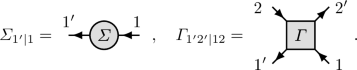(2)

These contain all one-particle irreducible one- and two-particle vertex diagrams, respectively. Hence, these are (amputated connected) diagrams that cannot be split into two pieces by cutting a single bare propagator line.

The one-particle self-energy is related to the two-particle vertex via the Schwinger–Dyson equation (SDE) . We do not discuss this equation much further because its treatment is similar for both vertex decompositions discussed below. On the two-particle level, the starting point of parquet approaches  is the parquet decomposition,

\begin{aligned} \varGamma = R + \gamma _a + \gamma _p + \gamma _t . \end{aligned}
(3)

It states that the set of all vertex diagrams can be divided into four disjoint classes: the diagrams in $$\gamma _r$$, $$r = a,p,t$$, are two-particle reducible in channel r, i.e., they can be split into two parts by cutting two antiparallel (a), parallel (p), or transverse antiparallel (t) propagator lines, respectively. The diagrams in R do not fall apart by cutting two propagator lines and are thus fully two-particle irreducible. This classification is exact and unambiguous [16, 38]. In the literature, the diagrammatic channels are also known as crossed particle–hole ($$\overline{\text {ph}}\leftrightarrow a$$), particle–particle (pp $$\leftrightarrow p$$), and particle–hole (ph $$\leftrightarrow t$$) channel.

Since the four classes in the parquet decomposition are disjoint, one can decompose $$\varGamma$$ w.r.t. its two-particle reducibility in one of the channels r, $$\varGamma = I_r + \gamma _r$$. Here, $$I_r$$ comprises the sum of all diagrams irreducible in channel r and fulfills $$I_r = R + \gamma _{{\bar{r}}}$$ with $$\gamma _{{\bar{r}}} = \sum _{r^\prime \ne r}\gamma _{r^\prime }$$. The Bethe–Salpeter equations (BSEs) relate the reducible diagrams to the irreducible ones and can be summarized by

\begin{aligned} \gamma _r = I_r \circ \varPi _r \circ \varGamma = \varGamma \circ \varPi _r \circ I_r. \end{aligned}
(4)

The $$\varPi _r$$ bubble, defined as

\begin{aligned} \varPi _{a;34|3'4'}&= G_{3|3'}G_{4|4'} , \end{aligned}
(5a)
\begin{aligned} \varPi _{p;34|3'4'}&= \tfrac{1}{2} G_{3|3'}G_{4|4'} , \end{aligned}
(5b)
\begin{aligned} \varPi _{t;43|3'4'}&= - G_{3|3'}G_{4|4'} , \end{aligned}
(5c)

represents the corresponding propagator pair in channel r, see Fig. 1. (Note that $$\varPi _{a;34|3'4'} = -\varPi _{t;43|3'4'}$$ is consistent with crossing symmetry.) The connector symbol $$\circ$$ denotes summation over internal frequencies and quantum numbers (5, 6 in Eqs. (6) below) and its definition depends on the channel $$r\in \{a,p,t\}$$: When connecting $$\varPi _r$$ (or other four-leg objects labeled by r) to some vertex, it gives

\begin{aligned} a: \quad [A \circ B]_{12|34}&= A_{ 16|54} B_{52|36}, \end{aligned}
(6a)
\begin{aligned} p: \quad [A \circ B]_{12|34}&= A_{ 12|56} B_{56|34}, \end{aligned}
(6b)
\begin{aligned} t: \quad [A \circ B]_{12|34}&= A_{ 62 | 54} B_{15|36}. \end{aligned}
(6c)

By combining $$\varGamma = I_r + \gamma _r$$ with the BSEs (4), one can eliminate $$\gamma _r$$ to get the “extended BSEs”  needed later:

\begin{aligned} \mathbbm {1}_r + \varPi _r\circ \varGamma&= (\mathbbm {1}_r-\varPi _r\circ I_r)^{-1}, \end{aligned}
(7a)
\begin{aligned} \mathbbm {1}_r + \varGamma \circ \varPi _r&= (\mathbbm {1}_r-I_r\circ \varPi _r)^{-1}. \end{aligned}
(7b)

Here, the channel-specific unit vertices $$\mathbbm {1}_r$$, defined by the requirement $$\varGamma = \mathbbm {1}_r\circ \varGamma = \varGamma \circ \mathbbm {1}_r$$, are given by

\begin{aligned} \mathbbm {1}_{a;12|34}&= \delta _{13}\delta _{24}, \end{aligned}
(8a)
\begin{aligned} \mathbbm {1}_{p;12|34}&= {\tfrac{1}{2}(\delta _{13}\delta _{24}-\delta _{14}\delta _{23})}, \end{aligned}
(8b)
\begin{aligned} \mathbbm {1}_{t;12|34}&= \delta _{14}\delta _{23}. \end{aligned}
(8c)

(For the p channel, the internal sum in $$\mathbbm {1}_p\circ \varGamma = \varGamma \circ \mathbbm {1}_p$$ runs over both outgoing (or ingoing) legs of $$\varGamma$$. Therefore, the crossing symmetry of the vertex, i.e., $$\varGamma _{12|34}=-\varGamma _{21|34}=-\varGamma _{12|43}$$, is transferred to $$\mathbbm {1}_p$$, resulting in an expression more involved than for the other two channels.)

The combination of the Dyson equation $$G = G_0(1 + \varSigma G)$$, the SDE, the parquet decomposition (3), the three BSEs (4), and the definitions $$I_r = \varGamma -\gamma _r$$ constitutes the self-consistent parquet equations. The only truly independent object is the fully irreducible vertex R. If R is specified, everything else can be computed self-consistently via the parquet equations. However, R is the most complicated object: its diagrams contain several nested integrals/sums over internal arguments, whereas the integrals in reducible diagrams partially factorize. A common simplification, the parquet approximation (PA), replaces R by U, closing the set of parquet equations.

### 2.2 Parquet mfRG

The conventional mfRG flow equations can be derived from the parquet equations by introducing a regulator $$\varLambda$$ into the bare propagator $$G_0$$, thus making all objects in the parquet equations $$\varLambda$$-dependent . The fully irreducible vertex R is treated as an input and is thus assumed to be $$\varLambda$$-independent, $$R^\varLambda \approx R$$. For instance, this assumption arises both in the PA where $$R \approx U$$ or in the dynamical vertex approximation D$$\varGamma$$A [39, 40] where $$R \approx R^{\mathrm {DMFT}}$$ is taken from DMFT—here, we will not distinguish these cases explicitly. Taking the derivative of the SDE and the BSEs w.r.t. $$\varLambda$$ then yields flow equations for $$\varSigma$$ and $$\varGamma$$. Within the context of this paper, we will call this mfRG approach parquet mfRG, to distinguish it from an SBE mfRG approach to be discussed in Sect. 3.2.

When computing $${\dot{\gamma }}_r = \partial _\varLambda \gamma _r$$ via the BSEs, one obtains terms including $${\dot{I}}_r = \sum _{r^\prime \ne r} {\dot{\gamma }}_{r^\prime }$$. Thus, one has to iteratively insert the flow equation for $$\gamma _r$$ into the equations of the other channels $$r'\ne r$$, yielding an infinite set of contributions of increasing loop order:

\begin{aligned} \dot{\varGamma } = \dot{\gamma }_a + \dot{\gamma }_p + \dot{\gamma }_t , \qquad \dot{\gamma }_r = \sum _{\ell =1}^\infty \dot{\gamma }_r^{(\ell )} . \end{aligned}
(9)

The individual $$\ell$$-loop contributions read [5, 7]

\begin{aligned} {\dot{\gamma }}_r^{(1)}&= \varGamma \circ {\dot{\varPi }}_r \circ \varGamma , \end{aligned}
(10a)
\begin{aligned} {\dot{\gamma }}_r^{(2)}&= {\dot{\gamma }}_{{{\bar{r}}}}^{(1)} \circ \varPi _r \circ \varGamma + \varGamma \circ \varPi _r \circ {\dot{\gamma }}_{{{\bar{r}}}}^{(1)} \end{aligned}
(10b)
\begin{aligned} {\dot{\gamma }}_r^{(\ell +2)}&= {\dot{\gamma }}_{{{\bar{r}}}}^{(\ell +1)} \circ \varPi _r \circ \varGamma \!+\! \varGamma \circ \varPi _r \circ {\dot{\gamma }}_{{{\bar{r}}}}^{(\ell )} \circ \varPi _r \circ \varGamma \nonumber \\&\quad \qquad + \varGamma \circ \varPi _r \circ {\dot{\gamma }}_{{{\bar{r}}}}^{(\ell +1)}. \end{aligned}
(10c)

where $$\dot{\gamma }_{\bar{r}}^{(\ell )} = \sum _{r'\ne r} \! \dot{\gamma }_{r'}^{(\ell )}$$ and Eq. (10c) applies for $$\ell +2 \ge 3$$. In general, all terms at loop order $$\ell$$ contain $$\ell -1$$ factors of $$\varPi$$ and one $${\dot{\varPi }}$$ (i.e., $$\ell$$ loops, one of which is differentiated), connecting $$\ell$$ renormalized vertices $$\varGamma$$. We have $${\dot{\varPi }}_r \sim G {\dot{G}} + {\dot{G}} G$$, where

\begin{aligned} {\dot{G}} = S + G \,\dot{\varSigma }\, G , \end{aligned}
(11)

with the single-scale propagator $$S = {\dot{G}} |_{\varSigma =\text {const}}$$. Figure 2 illustrates Eqs. (10) diagrammatically in the a channel.

The flow equation for the self-energy, derived in Ref.  by requiring $$\varSigma$$ to satisfy the SDE throughout the flow, reads(12)

It has $$\varGamma$$ and $${\dot{\gamma }}_{{\bar{t}},C}= \sum _\ell \varGamma \circ \varPi _r \circ {\dot{\gamma }}_{{{\bar{t}}}}^{(\ell )} \circ \varPi _r \circ \varGamma$$ as input and holds irrespective of the choice of vertex parametrization. For this reason, we do not discuss the self-energy flow further in this paper, but it should of course be implemented for numerical work.

The $$1\ell$$ contribution (10a) of the vertex flow, with the fully differentiated $$\dot{G}$$ replaced by the single-scale propagator S in $${\dot{\varPi }}_r$$ is equivalent to the usual $$1\ell$$ flow equation. Using $$\dot{G}$$ instead of S, as done in Eq. (10a), corresponds to the so-called Katanin substitution : it contains the feedback of the differentiated self-energy into the vertex flow and already goes beyond the standard $$1\ell$$ approximation. By adding higher-loop contributions until convergence is reached, one effectively solves the self-consistent parquet equations through an fRG flow. On the one hand, this ensures two-particle self-consistency and related properties mentioned in the introduction. On the other hand, it also provides a way of reaching a solution of the parquet equations by integrating differential equations. This may be numerically favorable compared to an iteration of the self-consistent equations. Particularly, when computing diagrammatic extensions of DMFT via $$\hbox {DMF}^2$$RG, one then needs only the full DMFT vertex as an input, and not the r-(ir)reducible ones entering the parquet equations. This is helpful in the Matsubara formalism, where the r-(ir)reducible vertices sometimes exhibit divergences [42,43,44,45,46], and even more so when aiming for real-frequency approaches [47, 48].

### 2.3 Frequency parametrization

The four-point vertex $$\varGamma$$ is a highly complicated object and must be parametrized efficiently. In this section, we summarize the frequency parametrization of the vertex adapted to the three diagrammatic channels. This parametrization is the building block for the SBE decomposition discussed in Sect. 3.

Focusing on the frequency dependence, we switch from the compact notation $$\varGamma _{1'2'|12}$$ to the more elaborate $$\varGamma _{1'2'|12} (\nu _1'\nu _2'|\nu _1\nu _2)$$, with frequency arguments written in brackets, and the subscripts now referring to non-frequency quantum numbers (position or momentum, spin, etc.). As mentioned earlier, we assume the bare vertex U to have the form

\begin{aligned} U_{1'2'|12} (\nu _1'\nu _2'|\nu _1\nu _2) =\delta _{\nu _1'+\nu _2',\nu _1+\nu _2} U_{1'2'|12} , \end{aligned}
(13)

with $$U_{1'2'|12}$$ independent of frequency. If U is momentum-conserving without further momentum dependence, our treatment of frequency sums below may be extended to include momentum sums. To keep the discussion general, we refrain from elaborating this in detail. Note that, e.g., in the repulsive Hubbard model, our sign convention in Eq. (1) is such that $$U^{\sigma {\bar{\sigma }}|\sigma {\bar{\sigma }}}=-U^{{\bar{\sigma }}\sigma |\sigma {\bar{\sigma }}}<0$$ (where, as usual, $$\sigma \in \{ \uparrow , \downarrow \}$$, $${\bar{\uparrow }} = \downarrow$$, $${\bar{\downarrow }} = \uparrow$$).

Due to frequency conservation, one-particle correlators depend on only one frequency,

\begin{aligned} G_{1'1}(\nu '_1,\nu _1) = \delta _{\nu '_1,\nu _1} G_{1'1}(\nu _1). \end{aligned}
(14)

Likewise, three frequencies are sufficient to parametrize the vertex. For each channel $$\gamma _r$$, we express the four fermionic frequencies $$\nu '_1, \nu '_2, \nu _1, \nu _2$$ at the vertex legs through a choice of three frequencies, a bosonic transfer frequency, $$\omega _r$$, and two fermionic frequencies, $$\nu _r$$ and $$\nu '_r$$. These are chosen differently for each channel (see Fig. 3a) and reflect its asymptotic behavior  as discussed in Sect. 4.1. We have

\begin{aligned}&\gamma _{r; 1'2'|12}(\nu _1'\nu _2'|\nu _1\nu _2) = \delta _{\nu _1'+\nu _2',\nu _1 + \nu _2} \gamma _{r;1'2'|12}(\omega _r,\nu _r,\nu _r') , \end{aligned}
(15)

with $$\omega _r$$, $$\nu _r$$, $$\nu _r'$$ related to $$\nu '_1$$, $$\nu _1$$, $$\nu _2$$ through

\begin{aligned} \nu '_1&= \nu _a- \tfrac{\omega _a}{2} = \nu _p+ \tfrac{\omega _p}{2} \,\,\,\,= \nu _t' + \tfrac{\omega _t}{2} , \nonumber \\ \nu _1&= \nu _a' - \tfrac{\omega _a}{2} = \nu _p' + \tfrac{\omega _p}{2}\,\,\,\, = \nu _t' - \tfrac{\omega _t}{2} , \nonumber \\ \nu _2&= \nu _a+ \tfrac{\omega _a}{2} = - \nu _p' + \tfrac{\omega _p}{2} = \nu _t+ \tfrac{\omega _t}{2} . \end{aligned}
(16)

This parametrization symmetrically assigns $$\pm \frac{\omega _r}{2}$$ shifts to all external legs. (In the Matsubara formalism, the bosonic Matsubara frequency closest to $$\pm \frac{\omega _r}{2}$$ is chosen for the shift.) With these shifts, crossing symmetries ensure that prominent vertex peaks are centered around $$\omega _r=0$$, which is convenient for numerical work. However, other conventions are of course possible, too.

Though the frequencies $$\omega _r, \nu _r, \nu _r'$$ are tailored to a specific channel $$\gamma _r$$, one may also use them to define the r parametrization of the full vertex, writing

\begin{aligned}&\varGamma _{1'2'|12}(\nu _1'\nu _2'|\nu _1\nu _2) = \delta _{\nu _1'+\nu _2',\nu _1 + \nu _2} \varGamma _{1'2'|12}(\omega _r,\nu _r,\nu _r') . &\end{aligned}
(17)

Likewise, $$R, \gamma _a, \gamma _p, \gamma _t$$ can each be expressed as a $$\delta$$ symbol times a function of any of the variable sets $$(\omega _r,\nu _r,\nu _r')$$. The r parametrization of $$\varGamma \circ \varPi _r$$ or $$\varPi _r \circ \varGamma$$ is obtained by inserting Eqs. (14) and (17) into Eqs. (6). The summations $$\sum _{\nu _5 \nu _6}$$ over internal frequencies can be collapsed using frequency-conserving $$\delta$$ symbols, leading to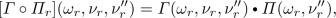(18a)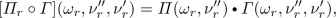(18b)

where the bubble factors $$\varPi _r (\omega _r, \nu ''_r)$$ are given by

\begin{aligned}&\varPi _{a;34|3'4'} (\omega _a, \nu ''_a) = G_{3|3'} \bigl (\nu ''_a \!-\! \tfrac{\omega _a}{2}\bigr ) G_{4|4'} \bigl (\nu ''_a \!+\! \tfrac{\omega _a}{2}\bigr ) , \end{aligned}
(19a)
\begin{aligned}&\varPi _{p;34|3'4'} (\omega _p, \nu ''_p) = \tfrac{1}{2} G_{3|3'} \bigl (\tfrac{\omega _p}{2} \!+\! \nu ''_p \bigr ) G_{4|4'} \bigl (\tfrac{\omega _p}{2} \!-\! \nu ''_p \bigr ) , \end{aligned}
(19b)
\begin{aligned}&\varPi _{t;43|3'4'} (\omega _t, \nu ''_t) = - G_{3|3'} \bigl (\nu ''_t \!-\! \tfrac{\omega _t}{2}\bigr ) G_{4|4'} \bigl (\nu ''_t \!+\! \tfrac{\omega _t}{2}\bigr ) . \end{aligned}
(19c)

In Eqs. (18), the connectorby definition denotes an internal summation analogous to $$\circ$$, except that only non-frequency quantum numbers (position, spin, etc.) are summed over. Correspondingly, the bubble $${\tilde{\varGamma }} \circ \varPi _r \circ \varGamma$$, involving two $$\circ$$ connectors, has the r parametrization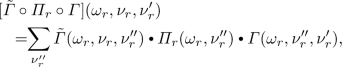(20)

see Fig. 3b. Here, one frequency sum survives, running over the fermionic frequency $$\nu ''_r$$ associated with $$\varPi _r$$.

For future reference, we define unit vertices for non-frequency quantum numbers, $${\mathbf {1}}_r$$, by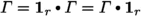. (For a bare vertex with momentum conservation and no further momentum dependence, one could include a momentum sum, $$\sum _{k''_r}$$, in Eq. (20) and exclude momentum indices from thesummation and $${\mathbf {1}}_r$$.) The distinction between $$\circ$$, $$\mathbbm {1}$$ and, $${\mathbf {1}}$$, indicating if connectors and unit vertices include summations and $$\delta$$ symbols for frequency variables or not, will be needed for the SBE decomposition of Sect. 3. There, we will encounter bubbles involving one or two bare vertices, $$U \circ \varPi _r \circ U$$, $${\tilde{\varGamma }} \circ \varPi _r \circ U$$, or $$U \circ \varPi _r \circ \varGamma$$. Expressing these in the form (20), the bare vertex U, since it is frequency independent, can be pulled out of the sum over $$\nu _r''$$. To make this explicit, we insert unit operators $${\mathbf {1}}_r$$ next to U: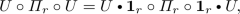(21a)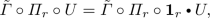(21b)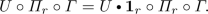(21c)

We suppressed frequency arguments for brevity, it being understood that equations linking $$\varPi _r$$ and $${\mathbf {1}}_r$$ use the r parametrization. Making the frequency sum involved in $$\circ \, \varPi _r \circ$$ explicit, we obtain four-leg objects,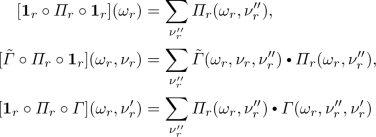(22)

that depend on only one or two frequency arguments (cf. Figure 3c) and are thus numerically cheaper than $$\varGamma$$. Note that, in general, $${\mathbf {1}}_r$$ is not the unit operator w.r.t. the $$\circ$$ connector, i.e., $${\mathbf {1}}_r \circ \varGamma \ne \varGamma \ne \varGamma \circ {\mathbf {1}}_r$$ since $$\circ$$ involves a frequency summation which does not affect $${\mathbf {1}}_r$$.

## 3 SBE decomposition

We now turn to the SBE decomposition. It also yields an exact, unambiguous classification of vertex diagrams, now according to their U-reducibility in each channel. This notion of reducibility, introduced in Ref. , is very analogous to $$\varPi$$-reducibility, i.e., two-particle reducibility. A diagram is called U-reducible if it can be split into two parts by splitting apart a bare vertex U (in ways specified below) in either of the three channels. Otherwise, it is fully U-irreducible.

The SBE decomposition was originally formulated in terms of physical (charge, spin, and singlet pairing) channels which involve linear combinations of spin components. For our purposes, it is more convenient not to use such linear combinations (the relation between both formulations is given in Appendix C). Moreover, the original SBE papers considered models with translational invariance, with vertices labeled by three momentum variables. We here present a generalization of the SBE decomposition applicable to models without translational invariance, requiring four position or momentum labels. Starting from the BSEs, we use arguments inspired by Ref.  to arrive at a set of self-consistent equations for SBE ingredients which will also enable us to derive multiloop flow equations directly within this framework. In terms of notation, we follow Ref.  for the objects $$\nabla _r$$, $$w_r$$, $${{\bar{\lambda }}}_r$$, $$\lambda _r$$—with $$\varphi ^{\text {firr}}$$ there denoted $$\varphi ^{U\text {irr}}$$ here—while we follow Ref.  for $$M_r$$ and $$T_r$$ (the latter instead of $$\varphi _r$$ from Ref. ).

### 3.1 Derivation of SBE decomposition from BSEs

As mentioned earlier, a vertex diagram is called two-particle reducible in a specified channel $$r \in \{a,p,t\}$$, or $$\varPi$$-r-reducible for short, if it can be split into two parts by cutting the two lines of a $$\varPi _r$$ bubble (to be called linking bubble); if such a split is not possible, the diagram is $$\varPi$$-r-irreducible. The two-particle reducible vertex $$\gamma _r$$ is the sum of all $$\varPi$$-r-reducible diagrams. Following Ref. , we now introduce a further channel-specific classification criterion. A $$\varPi$$-r-reducible diagram is called $$U{\hbox {-}}r$$-reducible if a linking bubble $$\varPi _r$$ has two of its legs attached to the same bare vertex in the combination $$U \circ \varPi _r$$ or $$\varPi _r \circ U$$. Then, that bare vertex U, too, constitutes a link that, when “cut out”, splits the diagram into two parts. (To visualize the meaning of “cutting out U” diagrammatically, one may replace U by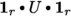and then remove U. This results in two pairs of legs ending close together, ready to be connected through reinsertion of U, see Figs. 3c and  4.) The lowest order $$U{\hbox {-}}r$$-reducible contribution to $$\gamma _r$$ is $$U \circ \varPi _r \circ U$$. The lowest-order term of $$\varGamma$$, the bare vertex U (which is $$\varPi$$-r-irreducible), is viewed as $$U{\hbox {-}}r$$-reducible in all three channels, corresponding to the three possible ways of splitting its four legs into two pairs of two. All $$U{\hbox {-}}r$$-reducible diagrams describe “single-boson exchange” processes, in the sense that each link U connecting two otherwise separate parts of the diagram mediates a single bosonic transfer frequency, $$\omega _r$$ (as defined in Fig. 3), across that link, as will become explicit below.

All vertex diagrams that are not $$U{\hbox {-}}r$$-reducible are called $$U{\hbox {-}}r$$-irreducible. These comprise all multi-boson exchange (i.e., not single-boson exchange) diagrams from $$\gamma _r$$, and all $$\varPi$$-r-irreducible diagrams except the bare vertex (which is trivially $$U{\hbox {-}}r$$-reducible), i.e., all diagrams from $$I_r-U = R-U + \sum _{r^\prime \ne r} \gamma _{r^\prime }$$.

Next, we rewrite the parquet equations in terms of $$U{\hbox {-}}r$$-reducible and $$U{\hbox {-}}r$$-irreducible parts. We define $$\nabla _r$$ as the sum of all $$U{\hbox {-}}r$$-reducible diagrams, including (importantly) the bare vertex U, and $$M_r$$ as the sum of all diagrams that are $$\varPi$$-r-reducible but $$U{\hbox {-}}r$$-irreducible, thus describing multi-boson exchange processes. Then, the $$\varPi$$-r-reducible vertex $$\gamma _r$$, which does not include U, fulfills

\begin{aligned} \gamma _r = \nabla _r - U + M_r . \end{aligned}
(23)

Inserting Eq. (23) for $$\gamma _r$$ into the parquet decomposition (3) yields

\begin{aligned} \varGamma&= \varphi ^{U\text {irr}}+{\textstyle \sum _r} \nabla _r-2U, \end{aligned}
(24a)
\begin{aligned} \varphi ^{U\text {irr}}&= R - U + {\textstyle \sum _r} M_r \ , \end{aligned}
(24b)

where $$\varphi ^{U\text {irr}}$$ is the fully U-irreducible part of $$\varGamma$$. The U subtractions ensure that the bare vertex U, which is contained once in each $$\nabla _r$$ but not in $$\varphi ^{U\text {irr}}$$, is not over-counted. Some low-order diagrams of $$\nabla _r$$, $$M_r$$, and R are shown in Fig. 5.

Just as $$\gamma _r$$, its parts $$\nabla _r$$ and $$M_r$$ satisfy Bethe–Salpeter-type equations, which we derive next. Inserting Eq. (23) into the full vertex $$\varGamma = I_r + \gamma _r$$, we split it into a $$U{\hbox {-}}r$$-reducible part, $$\nabla _r$$, and a $$U{\hbox {-}}r$$-irreducible remainder, $$T_r$$:

\begin{aligned} \varGamma&= \nabla _r + T_r , \end{aligned}
(25a)
\begin{aligned} T_r&= I_r - U + M_r . \end{aligned}
(25b)

The relation between the different decompositions of the full vertex implied by Eqs. (23)–(25) is illustrated in Fig. 6. Inserting Eqs. (23) and (25a) into either of the two forms of the BSEs (4) for $$\gamma _r$$, we obtain

\begin{aligned} \nabla _r - U + M_r&= I_r \circ \varPi _r \circ \nabla _r + I_r \circ \varPi _r \circ T_r \nonumber \\&= \nabla _r \circ \varPi _r \circ I_r + T_r \circ \varPi _r \circ I_r . \end{aligned}
(26)

This single set of equations can be split into two separate ones, one for $$\nabla _r -U$$, the other for $$M_r$$, containing only $$U{\hbox {-}}r$$-reducible or only $$U{\hbox {-}}r$$-irreducible terms, respectively. The first terms on the right are clearly $$U{\hbox {-}}r$$-reducible, since they contain $$\nabla _r$$. For the second terms on the right, we write $$I_r$$ as the sum of U and $$I_r - U$$, yielding $$U{\hbox {-}}r$$-reducible and $$U{\hbox {-}}r$$-irreducible contributions, respectively. We thus obtain two separate sets of equations,

\begin{aligned} \nabla _r - U&= I_r\circ \varPi _r \circ \nabla _r + U \circ \varPi _r \circ T_r \nonumber \\&= \nabla _r\circ \varPi _r \circ I_r + T_r \circ \varPi _r \circ U, \end{aligned}
(27)
\begin{aligned} M_r&= (I_r - U) \circ \varPi _r\circ T_r \nonumber \\&= T_r \circ \varPi _r \circ (I_r-U), \end{aligned}
(28)

the latter of which corresponds to Eq. (17) in Ref. . In Eqs. (27), we now bring all $$\nabla _r$$ contributions to the left,

\begin{aligned} (\mathbbm {1}_r - I_r \circ \varPi _r) \circ \nabla _r&= U \circ (\mathbbm {1}_r + \varPi _r \circ T_r) , \nonumber \\ \nabla _r \circ (\mathbbm {1}_r - \varPi _r \circ I_r )&= (\mathbbm {1}_r + T_r \circ \varPi _r ) \circ U, \end{aligned}
(29)

and solve for $$\nabla _r$$ by evoking the extended BSEs (7):

\begin{aligned} \nabla _r&= (\mathbbm {1}_r+\varGamma \circ \varPi _r)\circ U\circ (\mathbbm {1}_r+\varPi _r\circ T_r)\, \nonumber \\&= (\mathbbm {1}_r+T_r\circ \varPi _r)\circ U\circ (\mathbbm {1}_r+\varPi _r\circ \varGamma ). \end{aligned}
(30)

This directly exhibits the $$U{\hbox {-}}r$$-reducibility of $$\nabla _r$$.

We now adopt the r parametrization and note a key structural feature of Eq. (30) for $$\nabla _r$$: it contains a central bare vertex U, connected via $$\circ \, \varPi _r \, \circ$$ to either $$\varGamma$$ or $$T_r$$ or both. We may thus pull the frequency-independent U out of the frequency summations, so that $$\circ \, \varPi _r \, \circ$$ leads to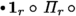or, where the multiplication with $${\mathbf {1}}_r$$ includes a sum over an internal fermionic frequency (recall Eqs. (21), (22) and Fig. 3). Thus, Eq. (30) leads to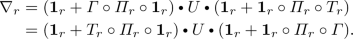(31)

In the first or second line, the expressions on the right or left of, respectively, are $$U{\hbox {-}}r$$-irreducible. These factors are the so-called Hedin vertices  (cf. Ref. , Eq. (5)),

\begin{aligned} {\bar{\lambda }}_r(\omega _r,\nu _r)&\equiv {\mathbf {1}}_r + [T_r \circ \varPi _r \circ {\mathbf {1}}_r] (\omega _r,\nu _r) , \end{aligned}
(32a)
\begin{aligned} \lambda _r(\omega _r,\nu _r')&\equiv {\mathbf {1}}_r + [ {\mathbf {1}}_r\circ \varPi _r \circ T_r ] (\omega _r,\nu _r') . \end{aligned}
(32b)

In our notation, the Hedin vertices have four fermionic legs, but (importantly) depend on only two frequencies. Indeed, regarding their frequency dependence, they can be viewed as the U-irreducible, amputated parts of three-point response functions (see Appendix D and Ref. ). Then, Eqs. (32) have the structure of SDEs for a three-point vertex with a bare three-point vertex $${\mathbf {1}}_r$$ (cf. Refs. [3, 7]). Via the Hedin vertices, $$\nabla _r$$ factorizes into functions of at most two frequency arguments and is thus computationally cheaper than, e.g., $$\gamma _r$$. Following Refs. [26, 30], we write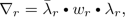(33)

where two $$U{\hbox {-}}r$$-irreducible Hedin vertices sandwich a $$U{\hbox {-}}r$$-reducible object, $$w_r(\omega _r)$$ (see Fig. 7). The object $$w_r$$ depends only on the bosonic frequency $$\omega _r$$ and can be interpreted as a screened interaction. To find $$w_r$$ explicitly, we first express Eq. (31) through Hedin vertices,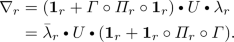(34)

Then, $$\varGamma = T_r + \nabla _r$$ leads to implicit relations for $$\nabla _r$$: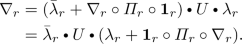(35)

Next, we insert Eq. (33) for $$\nabla _r$$ on both sides to obtain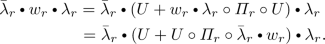(36)

This implies that $$w_r$$ satisfies a pair of Dyson equations,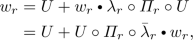(37)

which can be formally solved as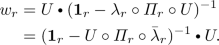(38)

As desired, the screened interaction $$w_r$$ is manifestly $$U{\hbox {-}}r$$-reducible, and depends on only a single, bosonic frequency, $$\omega _r$$. To emphasize this fact, Eq. (38) can be written as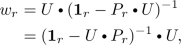(39)

where $$P_r (\omega _r)$$ is the polarization ,

\begin{aligned} P_r&= \lambda _r \circ \varPi _r \circ {\mathbf {1}}_r = {\mathbf {1}}_r \circ \varPi _r \circ {{\bar{\lambda }}}_r . \end{aligned}
(40)

Regarding frequency dependencies, $$w_r$$ can be viewed as a bosonic propagator and $$P_r$$ as a corresponding self-energy; Eq. (40) then has the structure of a SDE for $$P_r$$ involving the bare three-point vertex $${\mathbf {1}}_r$$ [3, 7].

Inserting Eq. (33) for $$\nabla _r$$ into Eq. (24a) for $$\varGamma$$, we arrive at the SBE decomposition of the full vertex of Ref.  in our generalized notation,(41a)

depicted diagrammatically in Fig. 8. For ease of reference, we gather all necessary relations for its ingredients: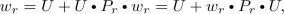(41b)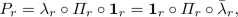(41c)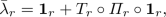(41d)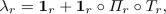(41e)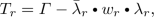(41f)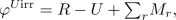(41g)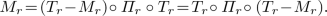(41h)

We collectively call Eqs. (41) the SBE equations. Together with the SDE for the self-energy and an input for the two-particle irreducible vertex R, the SBE equations are a self-consistent set of equations and thus fully define the four-point vertex $$\varGamma$$. They can either be solved self-consistently (as by Krien et al. in Refs. [27,28,29,30], where an analogous set of equations was set up), or via multiloop flow equations, derived in Sect. 3.2.

To conclude this section, let us point out the physical meaning of $${{\bar{\lambda }}}_r$$, $$w_r$$, $$\lambda _r$$ by showing their relation to three-point vertices and susceptibilities. For this, a symmetric expression for $$w_r$$ is needed, which can be obtained by comparing Eqs. (33) and (34) to deduce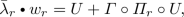(42a)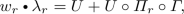(42b)

and inserting these into the Dyson equations (37):

\begin{aligned} w_r&= U + U\circ \varPi _r \circ U + U \circ \varPi _r\circ \varGamma \circ \varPi _r \circ U. \end{aligned}
(43)

Equations (42) and (43) can be expressed as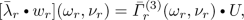(44a)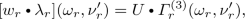(44b)(44c)

where $${{\bar{\varGamma }}}^{(3)}_r$$, $$\varGamma ^{(3)}_r$$ represent full three-point vertices and $$\chi _r$$ susceptibilities, defined by

\begin{aligned} {{\bar{\varGamma }}}^{(3)}_r(\omega _r,\nu _r)&= {\mathbf {1}}_r + [\varGamma \circ \varPi _r\circ {\mathbf {1}}_r](\omega ,\nu _r), \end{aligned}
(45a)
\begin{aligned} \varGamma ^{(3)}_r(\omega _r,\nu '_r)&= {\mathbf {1}}_r + [{\mathbf {1}}_r\circ \varPi _r\circ \varGamma ](\omega _r,\nu '_r), \end{aligned}
(45b)
\begin{aligned} \chi _r(\omega _r)&= [{\mathbf {1}}_r\circ \varPi _r\circ {\mathbf {1}}_r](\omega _r) \nonumber \\&\quad \quad + [{\mathbf {1}}_r\circ \varPi _r\circ \varGamma \circ \varPi _r\circ {\mathbf {1}}_r](\omega _r). \end{aligned}
(45c)

(The bare vertices were pulled out in front of the frequency sums, exploiting their frequency independence.) The relation of $${{\bar{\varGamma }}}^{(3)}_r$$ and $$\varGamma ^{(3)}_r$$ to three-point correlators and response functions is described in Appendix D; the relation of $$\chi _r$$ to physical susceptibilities for a local bare interaction U is discussed in Appendix E.

### 3.2 SBE mfRG from parquet mfRG

Having defined all the SBE ingredients, we are now ready to derive mfRG flow equations for them—the main goal of this work. Our strategy is to insert the SBE decomposition of Eqs. (23) and (24) into the parquet mfRG flow equations (10) for the $$\varPi$$-r-reducible vertices $$\gamma _r$$. An alternative derivation, starting directly from the SBE equations (41), is given in Sect. 3.3.

We begin by differentiating the decomposition of the $$\varPi _r$$-reducible vertex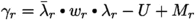(Eq. (23)) w.r.t. the flow parameter. Since $${\dot{U}}=0$$ (the bare vertex does not depend on the regulator), we obtain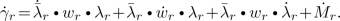(46)

The loop expansion $${\dot{\gamma }}_r = \sum _{\ell }{\dot{\gamma }}_{r}^{(\ell )}$$ implies similar expansions for $$\dot{w}_r$$, $$\dot{{{\bar{\lambda }}}}_r$$, $$\dot{\lambda }_r$$, and $$\dot{M}_r$$. Each term at a given loop order $$\ell$$ can be found from the mfRG flow (10) for $${\dot{\gamma }}_r^{(\ell )}$$, by inserting the decomposition of the full vertex,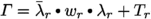(Eq. (25a)) on the right of Eqs. (10).

The $$1\ell$$ flow equation (10a) for $${\dot{\gamma }}_r^{(1)}$$ has four contributions (shown diagrammatically for $$\gamma _a^{(1)}$$ in Fig. 9):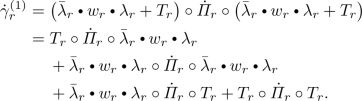(47)

By matching terms in Eqs. (46) and (47) containing factors of $${{\bar{\lambda }}}_r$$ and $$\lambda _r$$ or not, we obtain the $$1\ell$$ SBE flow: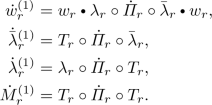(48a)

This reproduces the $$1\ell$$ SBE flow derived in Ref.  (their Eq. (18)). The higher loop terms can be found similarly from $${\dot{\gamma }}_{r}^{(2)}$$ and $${\dot{\gamma }}_{r}^{(\ell +2)}$$ of Eqs. (10b) and (10c). For each loop order $$\ell$$, the $$\dot{\gamma }_{{\bar{r}}}^{(\ell )}$$ factors on the right side of these equations can be expressed through the already known flow of $${\dot{w}}_{r'}^{(\ell )}$$, $$\dot{{{\bar{\lambda }}}}_{r'}^{(\ell )}$$ $${\dot{\lambda }}_{r'}^{(\ell )}$$ and $${\dot{M}}^{(\ell )}_{r'}$$. We obtain the flow equations ($$\ell +2 \ge 3$$)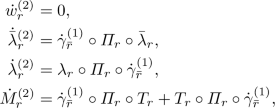(48b)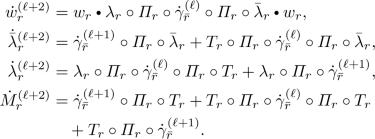(48c)

Here, $${\dot{\gamma }}_{{\bar{r}}}^{(\ell )}$$, required for the flow at loop orders $$\ell +1$$ and $$\ell +2$$, can directly be constructed from the SBE ingredients using Eq. (46). Similarly as in Eqs. (10), all terms at loop order $$\ell$$ contain $$\ell -1$$ factors of $$\varPi$$ and one $${\dot{\varPi }}$$, now connecting the renormalized objects $$w_r$$, $${{\bar{\lambda }}}_r$$, $$\lambda _r$$, $$T_r$$.

The SBE mfRG flow equations (48) are the most important result of this work. For the a channel, they are depicted diagrammatically in Fig. 10. Equations (48) can be condensed into more compact ones, giving the full flow (summed over all loop orders, $$\dot{w}_r = \sum _{\ell \ge 1} \dot{w}_r^{(\ell )}$$, etc.) of the SBE ingredients; see the next section. The multiloop flow equation for the self-energy [5, 7] is given in Eq. (12).

### 3.3 SBE mfRG from SBE equations

In the previous section, we derived the SBE mfRG flow equations by inserting the SBE decomposition into the known parquet mfRG flow equations of the two-particle reducible vertices $$\gamma _r$$. They can also be derived without prior knowledge on the flow of $$\gamma _r$$, using the techniques of Ref. .

In the parquet setting of Ref. , one can view the $$\varPi$$-r-irreducible vertex $$I_r$$ as the key ingredient for all equations related to channel r. In step (i), one uses $$I_r$$ to generate $$\gamma _r$$ and thus $$\varGamma$$ through a BSE. Then, a post-processing of attaching and closing external legs yields (ii) (full) three-point vertices $${{\bar{\varGamma }}}_r^{(3)}$$, $$\varGamma ^{(3)}_r$$ and (iii) a susceptibility $$\chi _r$$. The SBE setting can be understood in close analogy, with the only exception that one purposefully avoids generating U-r-reducible contributions, because these can (more efficiently) be constructed via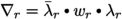. To exclude U-r-reducible contributions, one uses in step (i) $$I_r-U$$ to generate $$M_r$$ and thus $$T_r$$ through a BSE. The same post-processing as before yields (ii) $${{\bar{\lambda }}}_r$$, $$\lambda _r$$ and then (iii) $$w_r$$ or $$P_r$$.

Because of this structural analogy, the SBE mfRG flow equations can be derived in the exact same fashion as the parquet mfRG flow equation of Ref. . One merely has to replace the variables according to the dictionary

\begin{aligned} I_r&\rightarrow I_r - U, \quad \gamma _r \rightarrow M_r , \quad \varGamma \rightarrow T_r , \nonumber \\ {{\bar{\varGamma }}}^{(3)}_r&\rightarrow {{\bar{\lambda }}}_r , \quad \quad \varGamma ^{(3)}_r \rightarrow \lambda _r , \quad \chi _r \rightarrow P_r . \end{aligned}
(49)

For clarity, we now spell out the structural analogies between the original parquet formalism and its SBE version, presenting similarly-structured expressions in pairs of equations, (a) and (b). For both approaches, the full vertex can be decomposed in several ways:

\begin{aligned} \varGamma&= R + \sum _r \gamma _r = I_r + \gamma _r , \end{aligned}
(50a)
\begin{aligned} \varGamma&= \!R \!+\! \sum _r M_r \!+\! \sum _r (\nabla _r \!-\! U) \!=\! T_r + \nabla _r . \end{aligned}
(50b)

Here, $$\gamma _r$$ and $$M_r$$ satisfy analogous BSEs,

\begin{aligned} \gamma _r&= I_r \circ \varPi _r \circ \varGamma , \end{aligned}
(51a)
\begin{aligned} M_r&= (I_r-U) \circ \varPi _r \circ T_r , \end{aligned}
(51b)

where the objects on the left reappear on the right through

\begin{aligned} \varGamma&= I_r + \gamma _r , \end{aligned}
(52a)
\begin{aligned} T_r&= (I_r-U) + M_r . \end{aligned}
(52b)

Relations (51) and (52) are used for step (i). Differentiation of Eq. (51a) yields the mfRG flow of $$\dot{\gamma }_r$$ as in Eq. (10) and Fig. 2a of Ref. . Here, we replace the variables as above and start by differentiating Eq. (51b):

\begin{aligned} {\dot{M}}_r&= {\dot{I}}_r \circ \varPi _r \circ T_r + (I_r-U)\circ \dot{\varPi }_r\circ T_r\nonumber \\&\quad + (I_r-U)\circ \varPi _r\circ {\dot{I}}_r + (I_r-U)\circ \varPi _r\circ {\dot{M}}_r\nonumber \\ \Rightarrow {\dot{M}}_r&= (\mathbbm {1}_r-(I_r-U)\circ \varPi _r)^{-1}\circ \left[ {\dot{I}}_r \circ \varPi _r \circ T_r\right. \nonumber \\&\left. \quad + (I_r-U)\circ \dot{\varPi }_r\circ T_r + (I_r-U)\circ \varPi _r\circ {\dot{I}}_r\right] . \end{aligned}
(53)

For the first argument of Eq. (53), we used $$\partial _\varLambda (I_r - U) = {\dot{I}}_r$$, as $${\dot{U}}=0$$. Next, we use the extended BSE $$\mathbbm {1}_r + T_r\circ \varPi _r = \left( \mathbbm {1}_r-(I_r-U)\circ \varPi _r\right) ^{-1}$$ for $$M_r$$, cf. Eqs. (7) and (51). Recollecting the terms, we obtain the flow of $${\dot{M}}_r$$ as

\begin{aligned}&{\dot{M}}_r = T_r \circ {\dot{\varPi }}_r \circ T_r + {\dot{I}}_r \circ \varPi _r \circ T_r \nonumber \\&\qquad \quad + T_r \circ \varPi _r \circ {\dot{I}}_r \circ \varPi _r \circ T_r + T_r \circ \varPi _r \circ {\dot{I}}_r. \end{aligned}
(54)

A loop expansion with $$\dot{I}_r = {\dot{\gamma }}_{{{\bar{r}}}} = \sum _{\ell } {\dot{\gamma }}_{{\bar{r}}}^{(\ell )}$$ then yields our Eqs. (48) and Fig. 10.

For step (ii), we have the analogous relations

\begin{aligned} {\bar{\varGamma }}^{(3)}_r&= {\mathbf {1}}_r + \varGamma \circ \varPi _r \circ {\mathbf {1}}_r ,&\varGamma ^{(3)}_r&= {\mathbf {1}}_r + {\mathbf {1}}_r \circ \varPi _r \circ \varGamma , \end{aligned}
(55a)
\begin{aligned} {\bar{\lambda }}_r&= {\mathbf {1}}_r + T_r \circ \varPi _r \circ {\mathbf {1}}_r ,&\lambda _r&= {\mathbf {1}}_r + {\mathbf {1}}_r \circ \varPi _r \circ T_r . \end{aligned}
(55b)

Differentiation of Eq. (55a) yields the mfRG flow of $$\varGamma ^{(3)}_r$$ as in Eq. (42) and Fig. 7 of Ref. . Here, we again replace the variables as above and differentiate Eq. (55b):

\begin{aligned} \dot{{\bar{\lambda }}}_r&= {\dot{T}}_r \circ \varPi _r \circ {\mathbf {1}}_r + T_r \circ \dot{\varPi }_r\circ {\mathbf {1}}_r, \nonumber \\ \dot{\lambda }_r&= {\mathbf {1}}_r\circ \dot{\varPi }_r \circ T_r + {\mathbf {1}}_r\circ \varPi _r \circ {\dot{T}}_r. \end{aligned}
(56)

As $${\dot{T}}_r = {\dot{I}}_r + {\dot{M}}_r$$ (cf. Eq. (52b)), we insert the flow equation (54) for $${\dot{M}}_r$$ into Eq. (56) and use again Eq. (55b) This yields the flow equations

\begin{aligned} \dot{{\bar{\lambda }}}_r&= T_r \circ \dot{\varPi }_r \circ {{\bar{\lambda }}}_r + {\dot{I}}_r \circ \varPi _r \circ {{\bar{\lambda }}}_r + T_r \circ {\varPi }_r \circ {\dot{I}}_r \circ \varPi _r \circ {{\bar{\lambda }}}_r, \nonumber \\ \dot{\lambda }_r&= \lambda _r \circ \dot{\varPi }_r \circ T_r + \lambda _r \circ \varPi _r \circ {\dot{I}}_r + \lambda _r \circ \varPi _r \circ {\dot{I}}_r \circ \varPi _r \circ T_r. \end{aligned}
(57)

Their loop expansion reproduces Eqs. (48) and Fig. 10.

Finally, in step (iii), we have the relations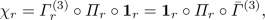(58a)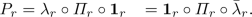(58b)

Differentiation of Eq. (58a) yields the mfRG flow of $$\chi _r$$ as in Eq. (44) and Fig. 8 of Ref. . Replacing the variables as above one more time, we differentiate Eq. (58b):

\begin{aligned} {\dot{P}}_r = {\mathbf {1}}_r\circ \varPi _r \circ \dot{{\bar{\lambda }}}_r + {\mathbf {1}}_r\circ \dot{\varPi }_r \circ {\bar{\lambda }}_r . \end{aligned}
(59)

After inserting Eqs. (55b) and (57), we eventually obtain

\begin{aligned} {\dot{P}}_r&= \lambda _r\circ \left( \dot{\varPi }_r+\varPi _r\circ {\dot{I}}_r\circ \varPi _r\right) \circ {{\bar{\lambda }}}_r . \end{aligned}
(60)

The relation between $${\dot{P}}_r$$ and $${\dot{w}}_r$$ follows from the Dyson equation (41b) as(61)

Solving this for $${\dot{w}}_r$$ yields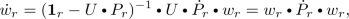(62)

having inserted the inverted Dyson equations (39). A loop expansion of Eq. (60) yields:

\begin{aligned} {\dot{P}}^{(1)}_r&= \lambda _r\circ \dot{\varPi }_r\circ {{\bar{\lambda }}}_r,\nonumber \\ {\dot{P}}^{(2)}_r&= 0,\nonumber \\ {\dot{P}}^{(\ell +2)}_r&= \lambda _r\circ \varPi _r\circ \dot{\gamma }_{{\bar{r}}}^{(\ell )}\circ \varPi _r\circ {{\bar{\lambda }}}_r. \end{aligned}
(63)

Inserting the loop expansion $${\dot{P}}_r^{(\ell )}$$ into Eq. (62) for $${\dot{w}}_r$$ yields the same flow equation for $$w_r$$ as in our Eqs. (48) and Fig. 10.

Depending on the specific model, it can be more efficient to calculate the flow of the polarization, $${\dot{P}}_r$$, by Eqs. (63) instead of the flow of the screened interaction, $${\dot{w}}_r$$, by Eqs. (48). The screened interaction on the contrary can be obtained by the inverted Dyson Eqs. (39).

Altogether, Eqs. (54), (57), (60) and (62) (with $$T_r$$ given by $$\varGamma -\nabla _{{\bar{r}}}$$, Eq. (50b)) build a system of closed fRG equations, as full derivatives of the SBE equations (41). Hence, combined with an appropriate self-energy flow (cf. Eq. (12) and Ref. ), they yield regulator-independent results. To integrate the flow equations in practice, one employs the mfRG loop expansions (48) and (63).

### 3.4 mfRG flow of the SBE approximation

To reduce numerical costs, it may sometimes be desirable to approximate the flow of the vertex treating only objects with less than all three frequency arguments. The simplest choice is to restrict the flow to functions depending on a single frequency. In the present context, this corresponds to keeping all objects except $$w_r$$ constant. With $$\dot{{{\bar{\lambda }}}}_r = 0 = {\dot{\lambda }}_r$$, the flow of the polarization (59) is simply

\begin{aligned} {\dot{P}}_r = \lambda _r\circ {\dot{\varPi }}_r\circ {\mathbf {1}}_r = {\mathbf {1}}_r\circ {\dot{\varPi }}_r\circ {{\bar{\lambda }}}_r. \end{aligned}
(64)

Hence, the flow equations of $$P_r$$ and $$w_r$$ completely decouple, and one effectively obtains a vertex consisting of three independent series of ladder diagrams. Nevertheless, such a flow may be helpful for code-developing purposes.

An approximation of the vertex with objects of at most two frequency arguments is given by the SBE approximation , which sets $$\varphi ^{U\text {irr}} = 0$$. More generally, one may also keep $$\varphi ^{U\text {irr}} \ne 0$$ constant during the flow, e.g., as obtained from DMFT (called SBE-D$$\varGamma$$A in Ref. ). This was used in a $$1\ell$$ implementation of $$\hbox {DMF}^2$$RG in Ref. . In the following, we will refer to the approximation of using a non-flowing U-irreducible part, $$\dot{\varphi }^{U\text {irr}}=0$$, as SBE approximation, regardless of whether $$\varphi ^{U\text {irr}}$$ is set to zero or not.

We now derive mfRG flow equations for the SBE approximation, so that $${\dot{R}}=0$$, as before, and furthermore $$\dot{M}_r = 0$$. For the most part, the SBE equations (41) remain unchanged. Only the BSE for $$M_r$$ (41h) is not considered anymore, since now $$\varphi ^{U\mathrm {irr}}=R-U+\sum _r M_r$$ is used as an input. The corresponding flow equations can be obtained as in Sect. 3.3. The flow of the polarization, the screened interaction and the Hedin vertices, prior to any transformation, is still given by Eqs. (59), (62) and (56) (collected here for convenience)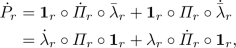(65a)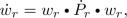(65b)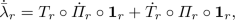(65c)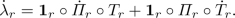(65d)

However, the flow of $$T_r = I_r - U + M_r$$ now has no $$\dot{M}_r$$ contribution. It is induced solely by $$\dot{I}_r = \dot{\nabla }_{{\bar{r}}}$$, the flow of the U-reducible contributions from complementary channels,

\begin{aligned} \dot{T}_r&= {\dot{\nabla }}_{{\bar{r}}}, \end{aligned}
(65e)

and thus is fully determined by $$\dot{{{\bar{\lambda }}}}_{{\bar{r}}}$$, $${\dot{\lambda }}_{{\bar{r}}}$$ and $$\dot{w}_{{\bar{r}}}$$.

Equations (65) can be rewritten by inserting the flow of the higher-point objects into the lower-point objects:

\begin{aligned}&\dot{{\bar{\lambda }}}_r = T_r\circ \dot{\varPi }_r\circ {\mathbf {1}}_r + \dot{\nabla }_{{\bar{r}}}\circ \varPi _r\circ {\mathbf {1}}_r, \end{aligned}
(66a)
\begin{aligned}&\dot{\lambda }_r = {\mathbf {1}}_r\circ \dot{\varPi }_r\circ T_r + {\mathbf {1}}_r\circ \varPi _r\circ \dot{\nabla }_{{\bar{r}}}, \end{aligned}
(66b)
\begin{aligned}&{\dot{P}}_r = {\mathbf {1}}_r\circ \dot{\varPi }_r \circ {\bar{\lambda }}_r + {\mathbf {1}}_r\circ \varPi _r \circ T_r \circ {\dot{\varPi }}_r \circ {\mathbf {1}}_r\nonumber \\&\qquad + {\mathbf {1}}_r\circ \varPi _r \circ \dot{\nabla }_{{\bar{r}}} \circ \varPi _r \circ {\mathbf {1}}_r\nonumber \\&\quad = {\mathbf {1}}_r\circ \dot{\varPi }_r \circ {\bar{\lambda }}_r + \lambda _r\circ {\dot{\varPi }}_r \circ {\mathbf {1}}_r\nonumber \\&\qquad -{\mathbf {1}}_r\circ {\dot{\varPi }}_r\circ {\mathbf {1}}_r + {\mathbf {1}}_r\circ \varPi _r \circ \dot{\nabla }_{{\bar{r}}} \circ \varPi _r \circ {\mathbf {1}}_r. \end{aligned}
(66c)

In the last line, we expressed $${\mathbf {1}}_r\circ \varPi _r\circ T_r$$ in terms of the Hedin vertex $$\lambda _r -{\mathbf {1}}_r$$. Equations (66) are similar to the previous flow equations (57) and (60) of the more general case, but some occurrences of the Hedin vertices $${{\bar{\lambda }}}_r, \lambda _r$$ on the right there are here replaced by their zeroth-order term $${\mathbf {1}}_r$$. Evidently, the contributions needed to upgrade these $${\mathbf {1}}_r$$ to $${{\bar{\lambda }}}_r, \lambda _r$$ are omitted when setting $$\dot{M}_r = 0$$.

A loop expansion of the above equations then yields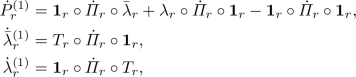(67a)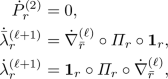(67b)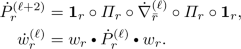(67c)

Apart from the fact that $$\dot{M}_r$$ is not needed here, the other flow equations are also simpler than Eqs. (48) without $$\dot{M}_r$$, obtained from the full SBE equations. To be specific, Eqs. (48) contain $${{\bar{\lambda }}}_r$$ or $$\lambda _r$$ on the right of the flow equations for $$\dot{{\bar{\lambda }}}_r^{(\ell )}$$ or $$\dot{{\lambda }}_r^{(\ell )}$$, whereas the simplified Eqs. (67) contain $${\mathbf {1}}_r$$ there, and, for $$\ell \ge 2$$, only one term where Eqs. (48) had two.

When using the above flow equations for the SBE approximation, the self-energy flow (12) should also be re-derived from either the SDE or the Hedin equation for $$\varSigma$$ (e.g. Eq. (23) in Ref. ). Since the present paper focuses on vertex parametrizations, we leave a derivation of a suitably modified self-energy flow for future work. Here, it suffices to note that, when used together with such a modified self-energy flow, Eqs. (67) are again total derivatives of a closed set of equations. So, integrating the flow until loop convergence would yield the regulator-independent solution of the SBE approximation.

Transforming the self-consistent equations of the SBE approximation on the vertex level to an equivalent mfRG flow reveals its simplistic nature, with relations like, and demonstrates how fRG offers an intuitive way to go beyond that, using, e.g.,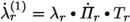(still treating only functions of at most two frequencies). However, the latter flow would be regulator-dependent per se. It remains to be seen how severe the lack of regulator independence for this flow, as used, e.g., in Ref. , is.

The simplified schemes presented in this section [i.e., Eqs. (64) and (67)] are closed flow equations on the vertex level and thus offer an appealing way for approaching the full SBE mfRG equations (48). Thereby, SBE ingredients with more complicated frequency dependence can be taken into account successively during code development. To what extent they can succeed in actually capturing the essential physics of a given problem will have to be investigated on a case-by-case basis. Generally, we showed that mfRG offers a way to make the choice of a certain approximation regulator independent, either for the simplistic flow of the SBE approximation or for the full SBE mfRG flow reproducing the PA.

## 4 Asymptotic classes

In numerical implementations of parquet mfRG [10,11,12,13,14], it is useful to handle the numerical complexity of the vertex by decomposing it into asymptotic classes with well-defined high-frequency behaviors. It is convenient to compute the flow of these asymptotic classes using their own flow equations; here, we recapitulate their derivation. We also elucidate the close relation between vertex parametrizations using the parquet decomposition with asymptotic classes or the SBE decomposition, deriving explicit equations relating their ingredients. These equations may facilitate the adaption of codes devised for parquet mfRG to SBE mfRG applications.

### 4.1 Definition of asymptotic classes

The parametrization of two-particle reducible vertices $$\gamma _r$$ via asymptotic classes was introduced in Ref.  to conveniently express their high-frequency asymptotics through simpler objects with fewer frequency arguments. One makes the ansatz

\begin{aligned}&\gamma _r(\omega _r,\nu _r,\nu _r') \nonumber \\&\quad \ = {\mathcal {K}}_{1}^{r}(\omega _r) \!+\! {\mathcal {K}}_{2}^{r}(\omega _r,\nu _r) \!+\! {\mathcal {K}}_{2^\prime }^{r}(\omega _r,\nu _r')\! +\! {\mathcal {K}}_{3}^{r}(\omega _r,\nu _r,\nu _r') . \end{aligned}
(68)

Here, $${\mathcal {K}}_{1}^{r}$$ contains all diagrams having both $$\nu _r$$ legs connected to the same bare vertex and both $$\nu _r'$$ legs connected to another bare vertex. (For a diagrammatic depiction, see Appendix B, Fig. 14.) These diagrams are thus independent of $$\nu _r$$, $$\nu _r'$$ and stay finite in the limit $$|\nu _r| \rightarrow \infty$$, $$|\nu _r'| \rightarrow \infty$$,

\begin{aligned} \lim _{|\nu _r| \rightarrow \infty } \ \lim _{|\nu _r'| \rightarrow \infty } \gamma _r(\omega _r,\nu _r,\nu _r') = {\mathcal {K}}_{1}^{r}(\omega _r). \end{aligned}
(69a)

$${\mathcal {K}}_{2}^{r}$$ (or $${\mathcal {K}}_{2^\prime }^{r}$$) analogously contains the part of the vertex having both $$\nu '_r$$ (or $$\nu _r$$) legs connected to the same bare vertex while the two $$\nu _r$$ (or $$\nu '_r$$) legs are connected to different bare vertices. Hence, it is finite for $$|\nu _r'| \rightarrow \infty$$ (or $$|\nu _r| \rightarrow \infty$$) but vanishes for $$|\nu _r| \rightarrow \infty$$ (or $$|\nu _r'| \rightarrow \infty$$):

\begin{aligned}&\lim _{|\nu _r'| \rightarrow \infty } \gamma _r(\omega _r,\nu _r,\nu _r') = {\mathcal {K}}_{1}^{r}(\omega _r) + {\mathcal {K}}_{2}^{r}(\omega _r, \nu _r) , \nonumber \\&\lim _{|\nu _r| \rightarrow \infty } \gamma _r(\omega _r,\nu _r,\nu _r') = {\mathcal {K}}_{1}^{r}(\omega _r) + {\mathcal {K}}_{2^\prime }^{r}(\omega _r, \nu _r') . \end{aligned}
(69b)

$${\mathcal {K}}_{3}^{r}$$ exclusively contains diagrams having both $$\nu _r$$ legs connected to different bare vertices, and likewise for both $$\nu '_r$$ legs. Such diagrams depend on all three frequencies and thus decay if any of them is sent to infinity. When taking the above limits for bubbles involving channels $$r'$$ different from r, we obtain zero,

\begin{aligned} \lim _{|\nu _r| \rightarrow \infty } \gamma _{r'\ne r} = \lim _{|\nu _r'| \rightarrow \infty } \gamma _{r'\ne r} = 0 , \end{aligned}
(69c)

as each $$\varPi _{r'}$$ in $$\gamma _{r'}$$ has a denominator containing $$\omega _{r'\ne r}$$, which is a linear combination of $$\omega _r$$, $$\nu _r$$ and $$\nu '_r$$.

Since R explicitly depends on all frequencies, it decays to the bare vertex U at high frequencies, and the asymptotic classes can be obtained by taking limits of the full vertex. Explicitly, $${\mathcal {K}}_{1}^{r}$$ can be obtained from

\begin{aligned}&\lim _{|\nu _r| \rightarrow \infty } \ \lim _{|\nu _r'| \rightarrow \infty } \varGamma (\omega _r,\nu _r,\nu _r') = U + {\mathcal {K}}_{1}^{r}(\omega _r), \end{aligned}
(70a)

taking the double limit in such a way that $$\nu _r \pm \nu _r'$$ is not constant, to ensure that all bosonic frequencies $$|\omega _{r' \ne r}|$$ go to $$\infty$$ . Similarly, $${\mathcal {K}}_{2}^{r}$$, $${\mathcal {K}}_{2^\prime }^{r}$$ can be obtained from objects $$\varGamma ^r_2$$, $$\varGamma ^r_{2'}$$ defined via the limits

\begin{aligned}&\varGamma ^r_2(\omega _r, \nu _r) \!\!=\!\! \lim _{|\nu _r'| \rightarrow \infty } \varGamma (\omega _r,\nu _r,\nu _r') \!=\! U + {\mathcal {K}}_{1}^{r} \!+\! {\mathcal {K}}_{2}^{r} , \end{aligned}
(70b)
\begin{aligned}&\varGamma ^r_{2'}(\omega _r, \nu _r') \!\!=\!\! \lim _{|\nu _r| \rightarrow \infty } \varGamma (\omega _r,\nu _r,\nu _r') \!=\! U + {\mathcal {K}}_{1}^{r} \!+\! {\mathcal {K}}_{2^\prime }^{r} . \end{aligned}
(70c)

For each of the latter two limits, we denote the complementary part of the vertex (vanishing in said limit) by

\begin{aligned}&{\bar{\varGamma }}^r_2(\omega _r, \nu _r, \nu _r') \!=\! \varGamma \!-\! \varGamma ^r_2= {\mathcal {K}}_{2^\prime }^{r} +{\mathcal {K}}_{3}^{r}+\gamma _{{\bar{r}}}\!+\!R \!-\! U , &\end{aligned}
(70d)
\begin{aligned}&{\bar{\varGamma }}^r_{2'}(\omega _r, \nu _r, \nu _r') \!=\! \varGamma \!-\! \varGamma ^r_{2'}= {\mathcal {K}}_{2}^{r}+{\mathcal {K}}_{3}^{r}+\gamma _{{\bar{r}}}\!+\!R \!-\! U . &\end{aligned}
(70e)

By taking suitable limits in the BSEs (4), the asymptotic classes can be expressed through the full vertex $$\varGamma$$ and the bare interaction U :

\begin{aligned} {\mathcal {K}}_{1}^{r}(\omega _r)&= U \circ (\varPi _r+\varPi _r \circ \varGamma \circ \varPi _r) \circ U, \end{aligned}
(71a)
\begin{aligned} {\mathcal {K}}_{2}^{r} (\omega _r,\nu _r)&= \varGamma \circ \varPi _r \circ U-{\mathcal {K}}_{1}^{r}, \end{aligned}
(71b)
\begin{aligned} {\mathcal {K}}_{2^\prime }^{r} (\omega _r,\nu _r')&= U \circ \varPi _r \circ \varGamma -{\mathcal {K}}_{1}^{r}. \end{aligned}
(71c)

Hence, they are directly related to the three-point vertices $${{\bar{\varGamma }}}^{(3)}_r$$, $$\varGamma ^{(3)}_r$$ and susceptibilities $$\chi _r$$ (cf. Eqs. (45) and Ref. ) as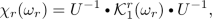(72a)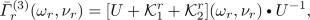(72b)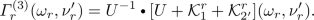(72c)

$${\mathcal {K}}_{1}^{r}$$ diagrams are therefore mediated by the bosonic fluctuations described by the susceptibility $$\chi _r$$, whereas $${\mathcal {K}}_{2}^{r}$$ and $${\mathcal {K}}_{2^\prime }^{r}$$ describe the coupling of fermions to these bosonic fluctuations via the three-point vertices $${{\bar{\varGamma }}}^{(3)}_r$$ and $$\varGamma ^{(3)}_r$$. This hints at the close relation between asymptotic classes and SBE components which is further discussed in Sec. 4.3.

### 4.2 mfRG equations for asymptotic classes

When the vertex is parametrized through its asymptotic classes, it is convenient to compute the latter directly during the flow, without numerically sending certain frequencies to infinity. This facilitates systematically adding or neglecting higher asymptotic classes. Therefore, we now derive explicit mfRG flow equations for the asymptotic classes, starting from the general multiloop flow equations (10), similar to the derivation of the mfRG flow equations for the SBE ingredients in Sect. 3.2. (For a diagrammatic derivation, see Refs. [50, 51].)

The parametrization (68) of $$\gamma _r$$ in terms of asymptotic classes holds analogously at each loop order,

\begin{aligned} {\dot{\gamma }}_{r}^{(\ell )} = \dot{{\mathcal {K}}}_{1}^{r(\ell )} + \dot{{\mathcal {K}}}_{2}^{r(\ell )} + \dot{{\mathcal {K}}}_{2'}^{r(\ell )} + \dot{{\mathcal {K}}}_{3}^{r(\ell )} . \end{aligned}
(73)

Then, each summand can be obtained from Eqs. (10) for $${\dot{\gamma }}_{r}^{(\ell )}$$ by taking suitable limits of the fermionic frequencies $$\nu _r, \nu '_r$$, as specified in Eqs. (69). For example, consider a bubble of type $$\varGamma \circ {\dot{\varPi }}_r \circ {\tilde{\varGamma }}$$, in the r representation of Eq. (20). In the limit $$|\nu _r|\rightarrow \infty$$, the first vertex reduces to $$\varGamma ^r_{2'}$$ (Eq. (70c)), while for $$|\nu '_r|\rightarrow \infty$$, the second vertex reduces to $${\tilde{\varGamma ^r_2}}$$ (Eq. (70b)). Using Eq. (20), we thus obtain

\begin{aligned} \lim _{|\nu _r|\rightarrow \infty }\varGamma \circ {\dot{\varPi }}_r\circ {\tilde{\varGamma }}&= \varGamma ^r_{2'}\circ {\dot{\varPi }}_r\circ {\tilde{\varGamma }}, \end{aligned}
(74a)
\begin{aligned} \lim _{|\nu '_r|\rightarrow \infty }\varGamma \circ {\dot{\varPi }}_r\circ {\tilde{\varGamma }}&= \varGamma \circ {\dot{\varPi }}_r\circ {\tilde{\varGamma ^r_2}}. \end{aligned}
(74b)

By contrast, when taking these limits for bubbles involving channels $$r'$$ different from r, we obtain zero,

\begin{aligned} \lim _{|\nu _r|\rightarrow \infty } \!\! \varGamma \circ {\dot{\varPi }}_{r' \ne r} \circ {\tilde{\varGamma }}&= 0 , \ \lim _{|\nu '_r|\rightarrow \infty } \!\! \varGamma \circ {\dot{\varPi }}_{r' \ne r} \circ {\tilde{\varGamma }} \, = 0 , &\end{aligned}
(74c)

by similar reasoning as that leading to Eq. (69c). In this manner, the $$1\ell$$ flow equation (10a) for $${\dot{\gamma }}_r^{(1)}$$ readily yields

\begin{aligned} \dot{{\mathcal {K}}}_{1}^{r\,(1)}&= \varGamma ^r_{2'}\circ \dot{\varPi }_r\circ \varGamma ^r_2, \nonumber \\ \dot{{\mathcal {K}}}_{2}^{r\,(1)}&= {\bar{\varGamma }}^r_{2'}\circ \dot{\varPi }_r\circ \varGamma ^r_2, \nonumber \\ \dot{{\mathcal {K}}}_{2^\prime }^{r\,(1)}&= \varGamma ^r_{2'}\circ \dot{\varPi }_r\circ {\bar{\varGamma }}^r_2, \nonumber \\ \dot{{\mathcal {K}}}_{3}^{r\,(1)}&= {\bar{\varGamma }}^r_{2'}\circ \dot{\varPi }_r\circ {\bar{\varGamma }}^r_2. \end{aligned}
(75a)

Similarly, the two-loop contribution $${\dot{\gamma }}_r^{(2)}$$, Eq. (10b), yields

\begin{aligned} \dot{{\mathcal {K}}}_{1}^{r\,(2)}&= 0 , \nonumber \\ \dot{{\mathcal {K}}}_{2}^{r\,(2)}&= \dot{\gamma }_{{\bar{r}}}^{(1)}\circ \varPi _r\circ \varGamma ^r_2, \nonumber \\ \dot{{\mathcal {K}}}_{2^\prime }^{r\,(2)}&= \varGamma ^r_{2'}\circ \varPi _r\circ \dot{\gamma }_{{\bar{r}}}^{(1)} , \nonumber \\ \dot{{\mathcal {K}}}_{3}^{r\,(2)}&= \dot{\gamma }_{{\bar{r}}}^{(1)}\circ \varPi _r\circ {\bar{\varGamma }}^r_2+{\bar{\varGamma }}^r_{2'}\circ \varPi _r\circ \dot{\gamma }_{{\bar{r}}}^{(1)} . \end{aligned}
(75b)

Due to Eq. (69c), $$\dot{{\mathcal {K}}}_{1}^{r\,(2)}$$ vanishes and $$\dot{{\mathcal {K}}}_{2}^{r\,(2)}$$ or $$\dot{{\mathcal {K}}}_{2'}^{r\,(2)}$$ contain no terms with $$\dot{\gamma }_{{\bar{r}}}^{(1)}$$ on their right or left sides, respectively. Finally, Eq. (10c) for $${\dot{\gamma }}_r^{(\ell +2)}$$, with $$\ell \ge 1$$, yields

\begin{aligned} \dot{{\mathcal {K}}}_{1}^{r\,(\ell + 2)}&= \varGamma ^r_{2'}\circ \varPi _r\circ \dot{\gamma }_{{\bar{r}}}^{(\ell )}\circ \varPi _r\circ \varGamma ^r_2, \nonumber \\ \dot{{\mathcal {K}}}_{2}^{r\,(\ell + 2)}&= \dot{\gamma }_{{\bar{r}}}^{(\ell +1)}\circ \varPi _r\circ \varGamma ^r_2+ {\bar{\varGamma }}^r_{2'}\circ \varPi _r\circ \dot{\gamma }_{{\bar{r}}}^{(\ell )}\circ \varPi _r\circ \varGamma ^r_2, \nonumber \\ \dot{{\mathcal {K}}}_{2^\prime }^{r\,(\ell + 2)}&= \varGamma ^r_{2'}\circ \varPi _r\circ \dot{\gamma }_{{\bar{r}}}^{(\ell )}\circ \varPi _r\circ {\bar{\varGamma }}^r_2+ \varGamma ^r_{2'}\circ \varPi _r\circ \dot{\gamma }_{{\bar{r}}}^{(\ell +1)}, \nonumber \\ \dot{{\mathcal {K}}}_{3}^{r\,(\ell + 2)}&= \dot{\gamma }_{{\bar{r}}}^{(\ell +1)}\circ \varPi _r\circ {\bar{\varGamma }}^r_2+ {\bar{\varGamma }}^r_{2'}\circ \varPi _r\circ \dot{\gamma }_{{\bar{r}}}^{(\ell )}\circ \varPi _r\circ {\bar{\varGamma }}^r_2\nonumber \\&\quad + {\bar{\varGamma }}^r_{2'}\circ \varPi _r\circ \dot{\gamma }_{{\bar{r}}}^{(\ell +1)} . \end{aligned}
(75c)

Here, $$\dot{{\mathcal {K}}}_{1}^{r(\ell + 2)} \ne 0$$ since $$\dot{\gamma }_{{\bar{r}}}^{(1)}$$ appears in the middle in the central term of Eq. (10c); hence, Eq. (69c) does not apply.

Note that these equations can also be used in the context of $$\hbox {DMF}^2$$RG [32, 33]. There, only the full vertex $$\varGamma$$ is given as an input. While $${\mathcal {K}}_{1}^{r}$$, $${\mathcal {K}}_{2}^{r}$$ and $${\mathcal {K}}_{2^\prime }^{r}$$ can be deduced from $$\varGamma$$ by sending certain frequencies to infinity (cf. Eqs. (70)) or using Eqs. (71), it is not possible to similarly extract $${\mathcal {K}}_{3}^{r}$$ in a given channel as some frequency limit of the full vertex $$\varGamma$$. However, the classes $${\mathcal {K}}_{3}^{r}$$ do not enter the right-hand sides of the flow equations (75) individually, but only the combination $$R+ {\mathcal {K}}_3 = R+\sum _r{\mathcal {K}}_{3}^{r}$$. This is already clear from the general formulation of the mfRG flow equations (10). Consider, e.g., the $$1\ell$$ contribution $$\dot{{\mathcal {K}}}_{2}^{r(1)}$$ of Eq. (75a). There, $${\bar{\varGamma }}^r_{2'}$$ contains $$R+ {\mathcal {K}}_{3}^{r} + \gamma _{{\bar{r}}} = R+ {\mathcal {K}}_3 + \sum _{r'\ne r} ({\mathcal {K}}_{1}^{r'} + {\mathcal {K}}_{2}^{r'} + {\mathcal {K}}_{2^\prime }^{r'})$$, and hence only requires knowledge of the full $$R + {\mathcal {K}}_3$$. This holds equivalently for all insertions of the full vertex into flow equations at any loop order. Now, insertions of the differentiated vertex in loop order $$\ell$$ into the flow equations of order $$\ell +1$$ and $$\ell +2$$ do require a channel decomposition $$\dot{{\mathcal {K}}}_3 = \sum _r\dot{{\mathcal {K}}}_{3}^{r}$$. For example, the two-loop contribution $$\dot{{\mathcal {K}}}_{2}^{r\,(2)}$$ of Eq. (75b) contains $$\dot{\gamma }_{{\bar{r}}}^{(1)}$$, which, by Eq. (73), involves differentiated vertices $$\dot{{\mathcal {K}}}_{3}^{r'\ne r \, (1)}$$. These are available via Eq. (75a). Therefore, in the $$\hbox {DMF}^2$$RG context, one would start with $${\mathcal {K}}_{1}^{r}$$, $${\mathcal {K}}_{2}^{r}$$, $${\mathcal {K}}_{2^\prime }^{r}$$ and the full $$R+ {\mathcal {K}}_{3}$$ from DMFT, compute the differentiated vertices $$\dot{{\mathcal {K}}}_{i}^{r}$$ independently (including $$\dot{{\mathcal {K}}}_{3}^{r}$$), successively insert them in higher loop orders, and eventually update $${\mathcal {K}}_{3}$$ using $$\dot{{\mathcal {K}}}_{3} = \sum _{\ell , r} \dot{{\mathcal {K}}}_{3}^{r \, (\ell )}$$ in each step of the flow (recall that R does not flow, $${\dot{R}}=0$$). The same reasoning also applies to the multi-boson terms $$M_r$$.

### 4.3 Relating SBE ingredients and asymptotic classes

The asymptotic classes and SBE ingredients are closely related . This is not surprising as the properties of both follow from the assumption that the bare vertex contains no frequency dependence, except for frequency conservation. For convenience, we collect these relations below.

Comparison of Eqs. (43) and (71a) yields

\begin{aligned} w_r(\omega _r)&= U + {\mathcal {K}}_{1}^{r}(\omega _r). \end{aligned}
(76)

Similarly, using Eqs. (42), (43), (71b), and (71c), we can write the products of Hedin vertices and the screened interaction as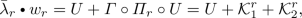(77a)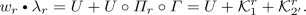(77b)

We now insert Eq. (76) for $$U \!+\! {\mathcal {K}}_{1}^{r}$$ and solve for $$\lambda _r$$, $${{\bar{\lambda }}}_r$$, formally defining $$w_r^{-1}$$ through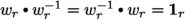. Thus, we obtain(78)

which, when inserted into Eq. (33), yields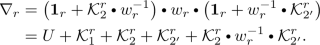(79)

Depending on model details, it may happen that not all components of $$w_r^{-1}$$ are uniquely defined. However, the right-hand sides of Eqs. (78)–(79) are unambiguous as the SBE ingredients are well defined through Eqs. (41).

Recalling that $$\gamma _r = \nabla _r - U + M_r$$, we conclude that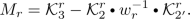(80)

Hence, $$\nabla _r$$ contains a part of $${\mathcal {K}}_{3}^{r}$$, namely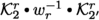, which can be fully expressed through functions that each depend on at most two frequencies. $$M_r$$ contains the remaining part of $${\mathcal {K}}_{3}^{r}$$, which must be explicitly parametrized through three frequencies and thus is numerically most expensive. A recent study of the Hubbard model showed that $$\sum _r M_r$$ is strongly localized in frequency space, particularly in the strong-coupling regime . This allows for a cheaper numerical treatment of the vertex part truly depending on three frequencies and constitutes the main computational advantage of the SBE decomposition.

Equations (76)–(79) fully express the SBE ingredients through asymptotic classes. Analogous results were obtained by similar arguments in Appendix A of Ref. . Figure 11 summarizes the relation between the two vertex decompositions and their ingredients.

Conversely, the asymptotic classes can also be expressed fully through the SBE ingredients. Using Eqs. (23), (68), (76), and (78), one finds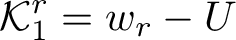(81a)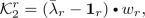(81b)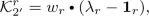(81c)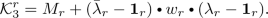(81d)

Moreover, Eqs. (25a), (70b), (70c), and (77) imply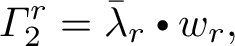(82a)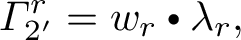(82b)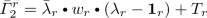(82c)(82d)

For the latter two equations, we used Eq. (25a) in the form. Equivalently, using the definitions of the Hedin vertices in Eqs. (32), we can express $${\mathcal {K}}_{2}^{r}$$, $${\mathcal {K}}_{3}^{r}$$, and Eqs. (82) as

\begin{aligned}&{\mathcal {K}}_{2}^{r} = T_r \circ \varPi _r \circ w_r , \end{aligned}
(83a)
\begin{aligned}&{\mathcal {K}}_{2^\prime }^{r} = w_r \circ \varPi _r \circ T_r , \end{aligned}
(83b)
\begin{aligned}&{\mathcal {K}}_{3}^{r} = M_r + T_r \circ \varPi _r \circ w_r \circ \varPi _r \circ T_r , \end{aligned}
(83c)
\begin{aligned}&\varGamma ^r_2= w_r + T_r \circ \varPi _r \circ w_r , \end{aligned}
(83d)
\begin{aligned}&\varGamma ^r_{2'}= w_r + w_r \circ \varPi _r \circ T_r , &\end{aligned}
(83e)
\begin{aligned}&{\bar{\varGamma }}^r_2= T_r + w_r \circ \varPi _r \circ T_r + T_r \circ \varPi _r \circ w_r \circ \varPi _r \circ T_r , \end{aligned}
(83f)
\begin{aligned}&{\bar{\varGamma }}^r_{2'}= T_r + T_r \circ \varPi _r \circ w_r + T_r \circ \varPi _r \circ w_r \circ \varPi _r \circ T_r . \end{aligned}
(83g)

Since the asymptotic classes and SBE ingredients are closely related, the same is true for their mfRG flow. Indeed, it is straightforward to derive the mfRG SBE flow equations (48) from the flow equations (75) for $$\dot{{\mathcal {K}}}_{i}^{r\,(\ell )}$$. We briefly indicate the strategy, without presenting all details.

We differentiate the equations (81) expressing $${\mathcal {K}}_{i}^{r}$$ through SBE ingredients, and subsequently use Eqs. (32) to eliminate $${\bar{\lambda }}_r-{\mathbf {1}}_r$$ and $${\lambda }_r-{\mathbf {1}}_r$$. Thereby, we obtain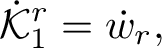(84a)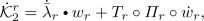(84b)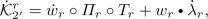(84c)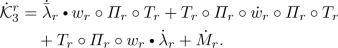(84d)

Now, we use Eqs. (75) to express the $$\dot{{\mathcal {K}}}_{i}^{r \, ({\ell })}$$ on the left through $$\varGamma ^r_2$$, $$\varGamma ^r_{2'}$$, $${\bar{\varGamma }}^r_2$$, $${\bar{\varGamma }}^r_{2'}$$, and Eqs. (82) to express the latter through SBE ingredients. By matching terms on the left and right in each loop order, we obtain flow equations for $$\dot{w}^{(\ell )}$$, $$\dot{{{\bar{\lambda }}}}_r^{(\ell )}$$, $${\dot{\lambda }}_r^{(\ell )}$$ and $$\dot{M}_r^{(\ell )}$$. For example, at $$1\ell$$ order, Eqs. (75a) and (84a) for $$\dot{{\mathcal {K}}}_{1}^{r \, (1)}$$ yield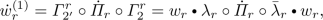(85)

consistent with Eq. (48a). Similarly, for $$\dot{{\mathcal {K}}}_{2}^{r \, (1)}$$, we obtain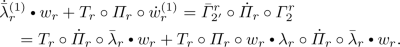(86)

The second terms on the left and right cancel due to Eq. (85). The remaining terms, right-multiplied by $$w_r^{-1}$$, yield $$\dot{{\bar{\lambda }}}_r^{(1)}= T_r \circ \dot{\varPi }_r\circ {\bar{\lambda }}_r$$, consistent with Eq. (48a). All of the equations (48) can be derived in this manner.

## 5 Conclusions and outlook

The SBE decomposition of the four-point vertex was originally introduced in Hubbard-like models respecting SU(2) spin symmetry and was written in terms of physical (e.g., spin and charge) channels . Inspired by Refs. [25,26,27,28,29,30], we here formulated the SBE decomposition without specifying the structure of non-frequency arguments (such as position or momentum, spin, etc.) starting from the parquet equations for general fermionic models. The only restriction on the structure of the bare vertex U is that, apart from being frequency-conserving, it is otherwise constant in frequency. Our formulation can thus be used as a starting point for a rather general class of models. It can also be easily extended to the Keldysh formalism or to other types of particles such as bosons or real fermions.

In this generalized framework, we re-derived self-consistent equations for the ingredients of the SBE decomposition, the so-called SBE equations, by separating the BSEs for the two-particle reducible vertices regarding their U-reducibility. The U-reducible $$\nabla _r$$ have a transparent interpretation through bosonic exchange fluctuations and Hedin vertices, describing the coupling of these bosonic fluctuations to fermions. As our main result, we derived multiloop flow equations for the SBE ingredients in two different ways: first by inserting the SBE decomposition into parquet mfRG and second by differentiating the SBE equations. Thereby, we presented the multiloop generalization of the $$1\ell$$ SBE flow of Ref. . In addition, we gave a detailed discussion of the relation between the SBE ingredients, $$M_r$$ and, and the asymptotic classes $${\mathcal {K}}_{i}^{r}$$ of the two-particle reducible vertices. Finally, we also presented multiloop flow equations for the $${\mathcal {K}}_{i}^{r}$$ and thus provided a unified formulation for the mfRG treatment of the parquet and the SBE vertex decompositions.

A numerical study of the SBE mfRG flow for relevant model systems, such as the single-impurity Anderson model or the Hubbard model, is left for future work. Below, we outline some open questions to be addressed.

The numerically most expensive SBE ingredient is the fully U-irreducible vertex $$\varphi ^{U\text {irr}}$$, involving the multi-boson exchange terms $$M_r$$, because these all depend on three frequency arguments. One may hope that, for certain applications, it might suffice to neglect $$\varphi ^{U\text {irr}}$$ (as done in Ref.  for a DMFT treatment of the Hubbard model), or to treat it in a cheap fashion, e.g., by not keeping track of its full frequency dependence or by not letting it flow (cf. Ref. ). This spoils the parquet two-particle self-consistency while retaining SBE self-consistency. It is an interesting open question which of the main qualitative features of the parquet solution, such as fulfillment of the Mermin–Wagner theorem , remain intact this way.

One formal feature, namely regulator independence, is maintained if multiloop flow equations in the SBE approximation are used. These equations are derived by setting $$\varphi ^{U\text {irr}}=0$$ and $$\dot{M}_r=0$$ from the beginning (Sect. 3.4) and are actually simpler than those obtained by setting $$\dot{M}_r=0$$ in the full SBE mfRG flow. We left the derivation of a self-energy flow directly within the SBE approximation for future work. The combination of such a self-energy flow with the vertex flow of Sect. 3.4 would constitute the total derivative of the SBE approximation. Therefore, if loop convergence can be achieved when integrating these simplified flow equations, the results will be regulator independent, just as for the full SBE mfRG flow with $$\varphi ^{U\text {irr}}=\sum _r M_r$$ and $$\dot{M}_r \ne 0$$, reproducing the PA.

Even if it turns out that a full treatment of $$\varphi ^{U\text {irr}}$$ is required for capturing essential qualitative features of the vertex, this might still be numerically cheaper than a full treatment of $${\mathcal {K}}_{3}^{}$$. The reason is that each $${\mathcal {K}}_{3}^{r}$$ contains a contribution, theterm in Eq. (79), which is included not in $$M_r$$ but in $$\nabla _r$$, and parametrized through the numerically cheaper Hedin vertices and screened interactions, see Fig. 11. If these terms decay comparatively slowly with frequency, their treatment via the $${\mathcal {K}}_{i}^{r}$$ decomposition would be numerically expensive, and the SBE decomposition could offer a numerically cheaper alternative. A systematic comparison of the numerical costs required to compute the multiloop flow of the two decompositions should thus be a main goal of future work.